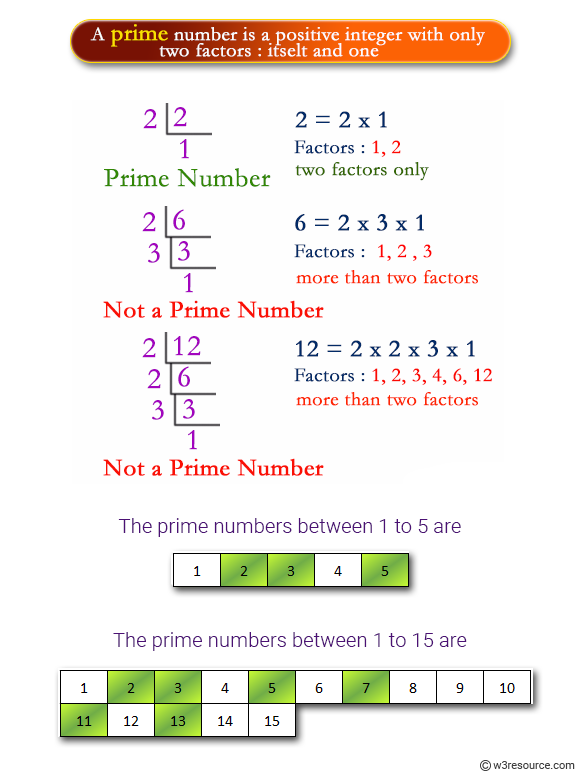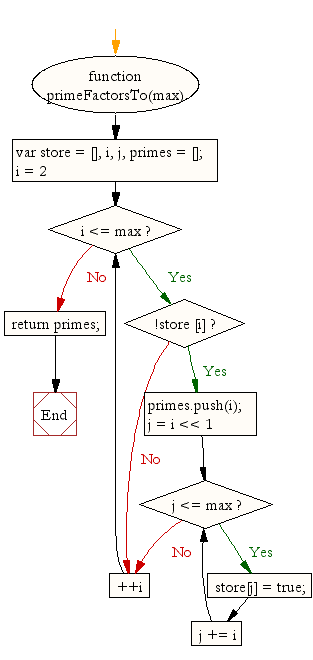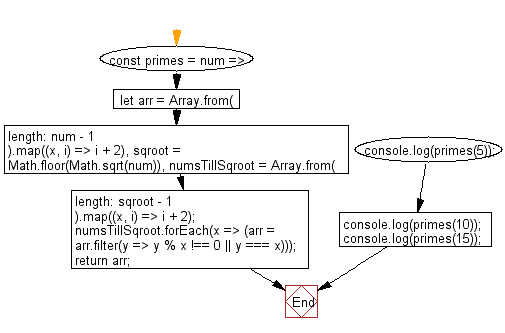# JavaScript: Get all prime numbers from 0 to a specified number

## JavaScript Math: Exercise-43 with Solution

Write a JavaScript function to get all prime numbers from 0 to a specified number

Test Data:
console.log(primeFactorsTo(5));
[2, 3, 5]
console.log(primeFactorsTo(15));
[2, 3, 5, 7, 11, 13]

Pictorial Presentation:Sample Solution-1:

JavaScript Code:

``````function primeFactorsTo(max)
{
var store  = [], i, j, primes = [];
for (i = 2; i <= max; ++i)
{
if (!store [i])
{
primes.push(i);
for (j = i << 1; j <= max; j += i)
{
store[j] = true;
}
}
}
return primes;
}

console.log(primeFactorsTo(5));

console.log(primeFactorsTo(15));
```
```

Sample Output:

```[2,3,5]
[2,3,5,7,11,13].
```

Flowchart:Sample Solution-2:

Generates primes up to a given number, using the Sieve of Eratosthenes.

• Generate an array from 2 to the given number.
• Use Array.prototype.filter() to filter out the values divisible by any number from 2 to the square root of the provided number.

JavaScript Code:

``````//Source:https://bit.ly/3hEZdCl
//primes
const primes = num => {
let arr = Array.from({ length: num - 1 }).map((x, i) => i + 2),
sqroot = Math.floor(Math.sqrt(num)),
numsTillSqroot = Array.from({ length: sqroot - 1 }).map((x, i) => i + 2);
numsTillSqroot.forEach(x => (arr = arr.filter(y => y % x !== 0 || y === x)));
return arr;
};

console.log(primes(5));
console.log(primes(10));
console.log(primes(15));
```
```

Sample Output:

```[2,3,5]
[2,3,5,7]
[2,3,5,7,11,13]
```

Flowchart:Live Demo:

See the Pen javascript-math-exercise-43 by w3resource (@w3resource) on CodePen.

Improve this sample solution and post your code through Disqus

What is the difficulty level of this exercise?

Test your Programming skills with w3resource's quiz.

﻿